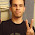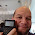## Sunday, December 16, 2012

### Adding Numbers and their Reverses

Please take a look at the Fun With Num3ers blog by Benjamin Vitale (Twitter: @BenVitale). This is a fun blog working with number theory. It is also an inspiration for me to blog. Thanks, Ben!

Eddie

On to today's blog entry!

Two Digit Numbers

Let AB represent a two digit number, where A and B each represent a digit 0 through 9 (excluding 00). My original goal was to find a number AB such that AB + BA = n where n contains both digits A and B. I was unsuccessful.

However, I did see something curious:

11 + 11 = 22
12 + 21 = 33
13 + 31 = 44
14 + 41 = 55
15 + 51 = 66
16 + 61 = 77
17 + 71 = 88
18 + 81 = 99
19 + 91 = 110
10 + 01 = 10 + 1 = 11

All the sums for this group are multiples of 11.

If I go on...
21 + 12 = 33
22 + 22 = 44
23 + 32 = 55
24 + 42 = 66
25 + 52 = 77
....

Once again, multiples of 11. Can we show that this is true for all sums AB + BA?

Let a represent the digit A. Similarly, let b represent the digit B. Then:

AB = (10a + b) and BA = (10b + a)

Then the sum AB + BA = (10a + b) + (10b + a) = 11a + 11b = 11(a + b)

Three Digit Numbers

Let's consider the number ABC. Rotate ABC one digit and add. Repeat. We get the sum ABC + BCA + CAB = n.

Let a, b, and c represent the digits A, B, and C, respectively. Then ABC = 100a + 10b + c. We can construct two similar sums for BCA and CAB.

Then

ABC + BCA + CAB
= (100a + 10b + c) + (100b + 10c + a) + (100c + 10a + b)
= 111a + 111b + 111c
= 111(a + b + c)

The sum is ends up a multiple of 111, but not necessarily of 11. (111 is not a multiple of 11). Examples?

Example 1:
425 + 254 + 542 = 1221
1221/111 = 11
1221/11 = 111

Example 2:
963 + 639 + 396 = 1998
1998/111 = 18
1998/11 = 181 6/11
1998 is not a multiple of 11

Can we find such triples ABC, BCA, and CAB such that the sum is divisible by both 11 and 111? Hint: the least common multiple of 11 and 111 is 1,221. I have found 31 such triplets (including the trivial 0,0,0).

If you want to find the triples, have fun! I'll leave you with a few examples:

29 + 902 + 290 = 1221. (029, 902, 290)
137 + 713 + 371 = 1221. (137, 713, 371)
598 + 859 + 985 = 2442. (598, 859, 985)
254 + 425 + 542 = 1221. (254, 425, 542)

Take care and be safe!

Eddie

This blog is property of Edward Shore. 2012

1.Notice that when you flip the digits of a number around and take the difference between them you always get a multiple of 9:

11-11 = 0
21-12 = 9
42-24 = 18
63-36 = 27
84-48 = 36

211-112 = 99
211-121 = 90

321-123 = 198 = 99*2 = 11*2*9
321-213 = 108 = 12*9
321-132 = 189 = 21*9
321-231 = 90
321-312 = 9

7546 - 4567 = 2979 = 331*9. There must be a way to prove this.

When you add a numer and it's reverse together, sometimes you get a palindrome:

11+11 = 22
21+12 = 33
65+56 = 121
123+321 = 444

Sometimes you have to do it more than once to reach a number that is a palindrome:

253+352 = 605
605+506 = 1111

143+431 = 574
574+475 = 1049
1049+9401 = 10450
10450+5401 = 15851

Somtimes you have to keep going, but you may never reach a palindrome. For example, add 89 and 98 together. Then add to that number it's reverse. Keep doing this until you reach a palindrome. I went ten or so iterations and I couldn't reach one!

2.Mike,

Let AB represent a two digit number, where a represents the digit A and b represents the digit B.

Then AB - BA implies that
(10a + b) - (10b + a) = 10a + b - 10b - a = 9(b - a)

Eddie

3.Hello Eddie !

Tanks a lot for your blog, i discovered it a few days ago ans really love it !

I've done a little program for my hp35s :

LBL U
0,99901
STO A
0
STO C
RCL A
IP
STO B
XEQ U028
XEQ U026
XEQ U026
+
+
10
*
11
RMDR
X<>0?
GTO U022
1
STO+C
ISG A
GTO U006
VIEW C
RTN
LASTx
IP
10
/
FP
RTN

In several minutes (about 7) : it gives 91
30 triplets + (000) = 31

Pascal

1.Pascal,

Thank you for the compliment. Nice program!

Eddie

### Calculator Python: Lambda Functions

Calculator Python: Lambda Functions Introduction to Lambda Functions Lambda functions are a quick, one expression, one line, python function...